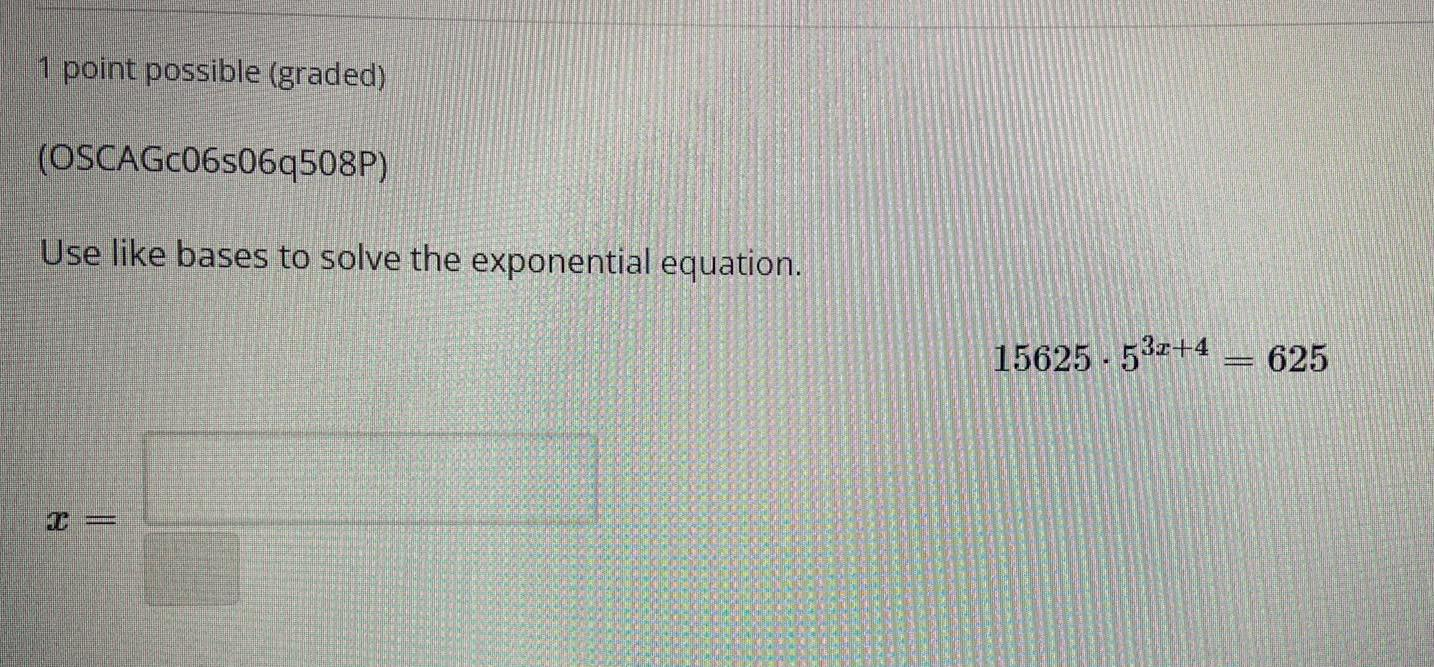Question:

# Use like bases to solve the exponential equation.

Last updated: 7/29/2022Use like bases to solve the exponential equation. 15625.5^(3x+4)=625 x=____